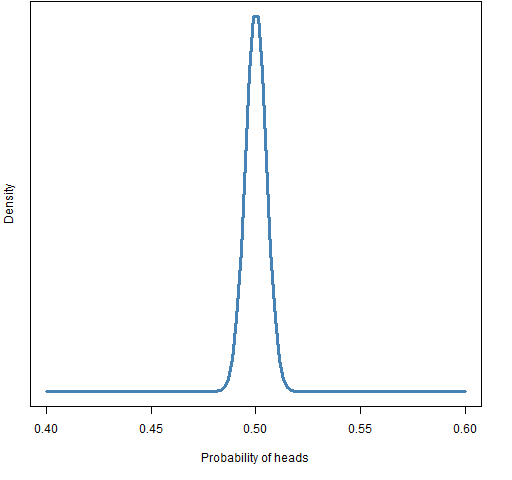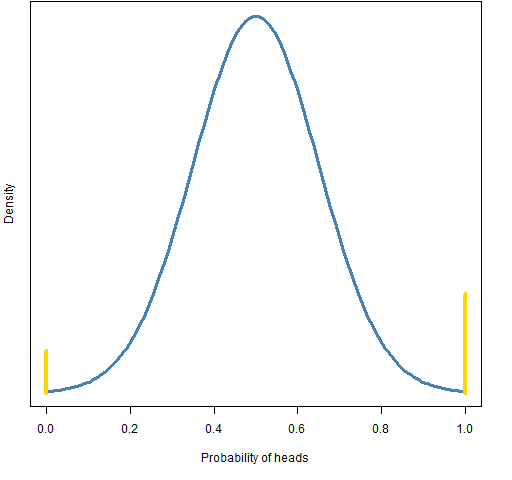Want to share your content on R-bloggers? click here if you have a blog, or here if you don't.

An introduction to Bayesian analysis and why you might care.

## Fight club

The subject of statistics is about how to learn.  Given that it is about the unknown, it shouldn’t be surprising that there are deep differences of opinion on how to go about doing it (in spite of the stereotype that statisticians are accountants minus the personality).

A slightly simplistic division of statistical philosophies is:

• frequentist estimator
• frequentist tester
• Bayesian

We’ll explore that division with the coin in my pocket.

## Flip a coin

What’s the probability — sight unseen — that a flip of my coin will be heads?

The frequentist estimator will say that without data there is no way to tell — it could be anything.  We’re in complete ignorance.

The frequentist tester will probably hypothesize that the probability is one-half, then say there is no evidence to reject that hypothesis.

A Bayesian will say that the probability has a distribution centered on one-half and is close to it.  Something like the distribution in Figure 1.

Figure 1: Bayesian prior distribution for the probability of “heads”.A smarter Bayesian will take account of whose pocket the coin is in, and say the distribution is more like that of Figure 2.

Figure 2: Alternative Bayesian prior for “heads”, point masses in gold.The coin could be two-headed, could be two-tailed, could be bent.

Figures 1 and 2 are prior distributions — what is thought before seeing any data.

## Learning

Now let’s start flipping the coin.

#### first flip

The first flip is heads.  That rules out that the probability is zero.

#### first 6 flips

The first 6 flips are all heads.

The frequentist estimator estimates that the probability is 1 with confidence intervals that go from 1 down to some value.  The 95% confidence interval goes down to 54%.

The frequentist tester may reject the hypothesis that the probability is one-half because the p-value is rather small.

The Bayesians will combine their priors with the data to arrive at a posterior distribution.  In this case these will look like the priors but will be shifted (somewhat) towards 1.  The posterior after 6 flips is the prior for flip 7.

#### first 100 flips

The frequentist estimator has a point estimate of 53% with a 95% confidence interval that goes from about 43% to 63%.

The frequentist tester will have to unreject the null hypothesis.

```R> binom.test(53, 100)

Exact binomial test

data:  53 and 100
number of successes = 53, number of trials =
100, p-value = 0.6173
alternative hypothesis: true probability of success is not equal to 0.5
95 percent confidence interval:
0.4275815 0.6305948
sample estimates:
probability of success
0.53```

The posterior for the first Bayesian will look fairly similar to the prior.  The posterior for the second Bayesian will look a whole lot like the posterior for the first.

## Statisticians in action

As you learned more about the coin in my pocket, you probably went through a process similar to the Bayesians (though you probably allowed the initial 6 heads to have too much impact).  The Bayesian way of thinking is more natural to a lot of people.

Yet Bayesian statistical analyses are in the minority.  Why?  Two reasons:

• computing power
• necessity

Bayesian analyses of more than textbook interest tend to require substantial computing power.  It is only recently that the requisite computing power has arrived.

For a lot of analyses, there is no need to be Bayesian.  If there is enough data, then the prior distribution makes essentially no difference at all.

Note 1: Frequentists will likely complain at this point that the real reason that frequentist analyses are predominant is because they are more valid.  Even conditional on that being true, I don’t think that is the reason for their ascendancy.  If frequentist analysis were computer intensive and Bayesian were not, then Bayesian would dominate.

Note 2: I consider myself a pragmatist.  I’m not particularly attached to any philosophy.

Fields like physics, agriculture and medicine can operate just fine without resorting to Bayesian statistics.  Mainly, at least — there may be cases where Bayes would be useful.

## Bayes goes to the bank

Finance is different.  While we have lots of numbers, we don’t necessarily have lots of information.

Finance is ripe for Bayesian statistics. We are often in the situation where our prior guesses do have a material effect on results.

But we don’t need to do a formal Bayesian analysis to benefit. The key element of Bayesian statistics is shrinkage.

After 6 coin flips we had all heads.  But our Bayesians didn’t believe the probability of heads was 1, that estimate was shrunk (somehow) towards 0.5.  (Real Bayesians resist producing estimates, they want to just give you their posterior.)

Here are a couple of examples of using shrinkage.

#### expected returns

If you have a model that predicts asset returns, it is not going to be perfect.  The Efficient Market Model says that the expected return is zero.  That model is not correct, but it’s pretty good.  You’ll be able to make your predictions better by shrinking them towards zero.  We have a prior that the expected returns are close to zero.

#### predicted variance matrix

Ledoit-Wolf variance estimation starts with the usual variance estimate and then shrinks it towards a simple model.  In particular, it shrinks towards all of the correlations being equal.  Thus we are using a prior that all the correlations are roughly equal.

## R for Bayes

The first stop for learning about Bayesian analyses in R should probably be the Bayesian task view.

Hot off the press is Stan which has an R interface.

Jarrod Wilcox has a piece on Bayesian investing.

You can learn more than you want to know at Wikipedia and Scholarpedia.

## Epilogue

Yo ye pharoahs, let us walk
Through this barren desert, in search of truth
And some pointy boots, and maybe a few snack crackers.

from “Camel Walk” by Rick Miller